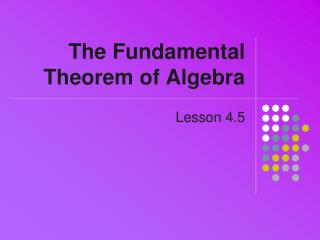DownloadDownload PresentationThe Fundamental Theorem of Algebra

# The Fundamental Theorem of Algebra

Download Presentation## The Fundamental Theorem of Algebra

- - - - - - - - - - - - - - - - - - - - - - - - - - - E N D - - - - - - - - - - - - - - - - - - - - - - - - - - -
##### Presentation Transcript

1. The Fundamental Theorem of Algebra Lesson 4.5

2. Example • Consider the solution to • Note the graph • No intersectionswith x-axis • Using the solve andcsolvefunctions

3. Fundamental Theorem of Algebra • A polynomial f(x) of degree n ≥ 1 has at least one complex zero • Remember that complex includes reals • Number of Zeros theorem • A polynomial of degree n has at most n distinct zeros • Explain how theorems apply to these graphs

4. Constructing a Polynomial with Prescribed Zeros • Given polynomial f(x) • Degree = 4 • Leading coefficient 2 • Zeros -3, 5, i, -i • Determine factored form • Determine expanded form

5. Conjugate Zeroes Theorem • Given a polynomial with real coefficients • If a + biis a zero, then a – bi will also be a zero

6. Finding Imaginary Zeros • Given • Determine all zeros • Use calculator to factor • Try cFactor command • Use calculator to graph • Use cSolve or cZeros

7. Application • Complex numbers show up in study of electrical circuits • Impedance, Z • Voltage, V Can be represented by • Current, I complex numbers • Find missing value

8. Assignment • Lesson 4.5 • Page 307 • Exercises 1 – 41 EOO 43 – 47 odd